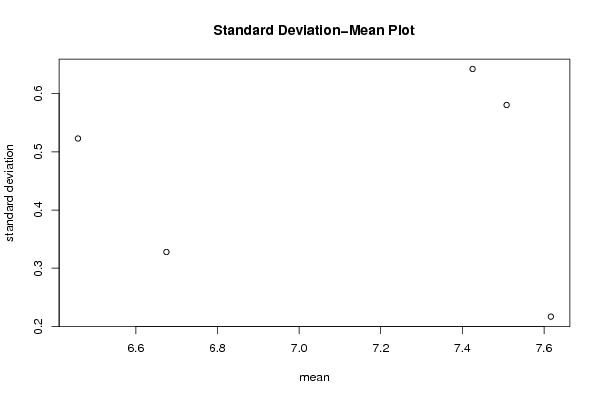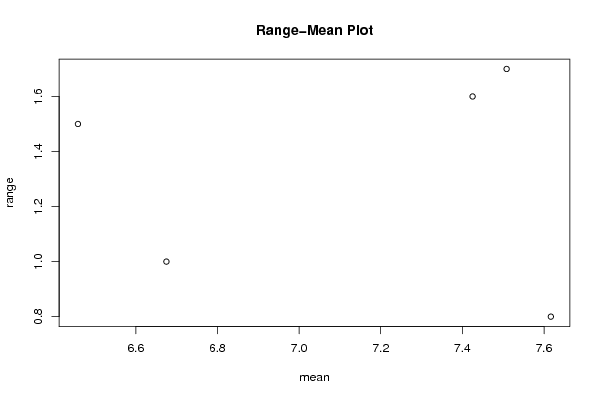## Free Statistics

of Irreproducible Research!

Author's title
Author*The author of this computation has been verified*
R Software Modulerwasp_smp.wasp
Title produced by softwareStandard Deviation-Mean Plot
Date of computationTue, 24 Nov 2009 11:38:42 -0700
Cite this page as followsStatistical Computations at FreeStatistics.org, Office for Research Development and Education, URL https://freestatistics.org/blog/index.php?v=date/2009/Nov/24/t125908808479hwl93nxy3g59j.htm/, Retrieved Tue, 05 Dec 2023 22:47:21 +0000
Statistical Computations at FreeStatistics.org, Office for Research Development and Education, URL https://freestatistics.org/blog/index.php?pk=59217, Retrieved Tue, 05 Dec 2023 22:47:21 +0000
QR Codes:Original text written by user:
IsPrivate?No (this computation is public)
User-defined keywords
Estimated Impact242
Family? (F = Feedback message, R = changed R code, M = changed R Module, P = changed Parameters, D = changed Data)
-     [Univariate Explorative Data Analysis] [Run Sequence gebo...] [2008-12-12 13:32:37] [76963dc1903f0f612b6153510a3818cf]
- R  D  [Univariate Explorative Data Analysis] [Run Sequence gebo...] [2008-12-17 12:14:40] [76963dc1903f0f612b6153510a3818cf]
-         [Univariate Explorative Data Analysis] [Run Sequence Plot...] [2008-12-22 18:19:51] [1ce0d16c8f4225c977b42c8fa93bc163]
- RMP       [Standard Deviation-Mean Plot] [Identifying Integ...] [2009-11-22 12:50:05] [b98453cac15ba1066b407e146608df68]
-    D          [Standard Deviation-Mean Plot] [Heterokedasticity] [2009-11-24 18:38:42] [acc980be4047884b6edd254cd7beb9fa] [Current]
- R               [Standard Deviation-Mean Plot] [] [2009-12-04 21:26:53] [859f65298c93b90426725427c75f8582]
-                 [Standard Deviation-Mean Plot] [] [2009-12-13 10:48:15] [b7349fb284cae6f1172638396d27b11f]
Feedback Forum

Post a new message
Dataseries X:
8.2
8
7.5
6.8
6.5
6.6
7.6
8
8.1
7.7
7.5
7.6
7.8
7.8
7.8
7.5
7.5
7.1
7.5
7.5
7.6
7.7
7.7
7.9
8.1
8.2
8.2
8.2
7.9
7.3
6.9
6.6
6.7
6.9
7
7.1
7.2
7.1
6.9
7
6.8
6.4
6.7
6.6
6.4
6.3
6.2
6.5
6.8
6.8
6.4
6.1
5.8
6.1
7.2
7.3
6.9
6.1
5.8
6.2
7.1
7.7
7.9
7.7
7.4
7.5
8
8.1
8

 Summary of computational transaction Raw Input view raw input (R code) Raw Output view raw output of R engine Computing time 1 seconds R Server 'Gwilym Jenkins' @ 72.249.127.135

\begin{tabular}{lllllllll}
\hline
Summary of computational transaction \tabularnewline
Raw Input & view raw input (R code)  \tabularnewline
Raw Output & view raw output of R engine  \tabularnewline
Computing time & 1 seconds \tabularnewline
R Server & 'Gwilym Jenkins' @ 72.249.127.135 \tabularnewline
\hline
\end{tabular}
%Source: https://freestatistics.org/blog/index.php?pk=59217&T=0

[TABLE]
[ROW][C]Summary of computational transaction[/C][/ROW]
[ROW][C]Raw Input[/C][C]view raw input (R code) [/C][/ROW]
[ROW][C]Raw Output[/C][C]view raw output of R engine [/C][/ROW]
[ROW][C]Computing time[/C][C]1 seconds[/C][/ROW]
[ROW][C]R Server[/C][C]'Gwilym Jenkins' @ 72.249.127.135[/C][/ROW]
[/TABLE]
Source: https://freestatistics.org/blog/index.php?pk=59217&T=0

Globally Unique Identifier (entire table): ba.freestatistics.org/blog/index.php?pk=59217&T=0

As an alternative you can also use a QR Code:

The GUIDs for individual cells are displayed in the table below:

 Summary of computational transaction Raw Input view raw input (R code) Raw Output view raw output of R engine Computing time 1 seconds R Server 'Gwilym Jenkins' @ 72.249.127.135

 Standard Deviation-Mean Plot Section Mean Standard Deviation Range 1 7.50833333333333 0.580686636402948 1.7 2 7.61666666666667 0.216724933890166 0.8 3 7.425 0.642615394660403 1.6 4 6.675 0.3278719262151 1 5 6.45833333333333 0.523030215246315 1.5

\begin{tabular}{lllllllll}
\hline
Standard Deviation-Mean Plot \tabularnewline
Section & Mean & Standard Deviation & Range \tabularnewline
1 & 7.50833333333333 & 0.580686636402948 & 1.7 \tabularnewline
2 & 7.61666666666667 & 0.216724933890166 & 0.8 \tabularnewline
3 & 7.425 & 0.642615394660403 & 1.6 \tabularnewline
4 & 6.675 & 0.3278719262151 & 1 \tabularnewline
5 & 6.45833333333333 & 0.523030215246315 & 1.5 \tabularnewline
\hline
\end{tabular}
%Source: https://freestatistics.org/blog/index.php?pk=59217&T=1

[TABLE]
[ROW][C]Standard Deviation-Mean Plot[/C][/ROW]
[ROW][C]Section[/C][C]Mean[/C][C]Standard Deviation[/C][C]Range[/C][/ROW]
[ROW][C]1[/C][C]7.50833333333333[/C][C]0.580686636402948[/C][C]1.7[/C][/ROW]
[ROW][C]2[/C][C]7.61666666666667[/C][C]0.216724933890166[/C][C]0.8[/C][/ROW]
[ROW][C]3[/C][C]7.425[/C][C]0.642615394660403[/C][C]1.6[/C][/ROW]
[ROW][C]4[/C][C]6.675[/C][C]0.3278719262151[/C][C]1[/C][/ROW]
[ROW][C]5[/C][C]6.45833333333333[/C][C]0.523030215246315[/C][C]1.5[/C][/ROW]
[/TABLE]
Source: https://freestatistics.org/blog/index.php?pk=59217&T=1

Globally Unique Identifier (entire table): ba.freestatistics.org/blog/index.php?pk=59217&T=1

As an alternative you can also use a QR Code:

The GUIDs for individual cells are displayed in the table below:

 Standard Deviation-Mean Plot Section Mean Standard Deviation Range 1 7.50833333333333 0.580686636402948 1.7 2 7.61666666666667 0.216724933890166 0.8 3 7.425 0.642615394660403 1.6 4 6.675 0.3278719262151 1 5 6.45833333333333 0.523030215246315 1.5

 Regression: S.E.(k) = alpha + beta * Mean(k) alpha 0.464650977406539 beta -0.000905906976677215 S.D. 0.195137943476804 T-STAT -0.00464239276348067 p-value 0.996587369311516

\begin{tabular}{lllllllll}
\hline
Regression: S.E.(k) = alpha + beta * Mean(k) \tabularnewline
alpha & 0.464650977406539 \tabularnewline
beta & -0.000905906976677215 \tabularnewline
S.D. & 0.195137943476804 \tabularnewline
T-STAT & -0.00464239276348067 \tabularnewline
p-value & 0.996587369311516 \tabularnewline
\hline
\end{tabular}
%Source: https://freestatistics.org/blog/index.php?pk=59217&T=2

[TABLE]
[ROW][C]Regression: S.E.(k) = alpha + beta * Mean(k)[/C][/ROW]
[ROW][C]alpha[/C][C]0.464650977406539[/C][/ROW]
[ROW][C]beta[/C][C]-0.000905906976677215[/C][/ROW]
[ROW][C]S.D.[/C][C]0.195137943476804[/C][/ROW]
[ROW][C]T-STAT[/C][C]-0.00464239276348067[/C][/ROW]
[ROW][C]p-value[/C][C]0.996587369311516[/C][/ROW]
[/TABLE]
Source: https://freestatistics.org/blog/index.php?pk=59217&T=2

Globally Unique Identifier (entire table): ba.freestatistics.org/blog/index.php?pk=59217&T=2

As an alternative you can also use a QR Code:

The GUIDs for individual cells are displayed in the table below:

 Regression: S.E.(k) = alpha + beta * Mean(k) alpha 0.464650977406539 beta -0.000905906976677215 S.D. 0.195137943476804 T-STAT -0.00464239276348067 p-value 0.996587369311516

 Regression: ln S.E.(k) = alpha + beta * ln Mean(k) alpha 0.434869833998904 beta -0.657413393444926 S.D. 3.46721317435426 T-STAT -0.189608587757908 p-value 0.86171942092768 Lambda 1.65741339344493

\begin{tabular}{lllllllll}
\hline
Regression: ln S.E.(k) = alpha + beta * ln Mean(k) \tabularnewline
alpha & 0.434869833998904 \tabularnewline
beta & -0.657413393444926 \tabularnewline
S.D. & 3.46721317435426 \tabularnewline
T-STAT & -0.189608587757908 \tabularnewline
p-value & 0.86171942092768 \tabularnewline
Lambda & 1.65741339344493 \tabularnewline
\hline
\end{tabular}
%Source: https://freestatistics.org/blog/index.php?pk=59217&T=3

[TABLE]
[ROW][C]Regression: ln S.E.(k) = alpha + beta * ln Mean(k)[/C][/ROW]
[ROW][C]alpha[/C][C]0.434869833998904[/C][/ROW]
[ROW][C]beta[/C][C]-0.657413393444926[/C][/ROW]
[ROW][C]S.D.[/C][C]3.46721317435426[/C][/ROW]
[ROW][C]T-STAT[/C][C]-0.189608587757908[/C][/ROW]
[ROW][C]p-value[/C][C]0.86171942092768[/C][/ROW]
[ROW][C]Lambda[/C][C]1.65741339344493[/C][/ROW]
[/TABLE]
Source: https://freestatistics.org/blog/index.php?pk=59217&T=3

Globally Unique Identifier (entire table): ba.freestatistics.org/blog/index.php?pk=59217&T=3

As an alternative you can also use a QR Code:

The GUIDs for individual cells are displayed in the table below:

 Regression: ln S.E.(k) = alpha + beta * ln Mean(k) alpha 0.434869833998904 beta -0.657413393444926 S.D. 3.46721317435426 T-STAT -0.189608587757908 p-value 0.86171942092768 Lambda 1.65741339344493PNG link Postscript link PDF linkPNG link Postscript link PDF link

Parameters (Session):
par1 = 12 ;
Parameters (R input):
par1 = 12 ;
R code (references can be found in the software module):
par1 <- as.numeric(par1)(n <- length(x))(np <- floor(n / par1))arr <- array(NA,dim=c(par1,np))j <- 0k <- 1for (i in 1:(np*par1)){j = j + 1arr[j,k] <- x[i]if (j == par1) {j = 0k=k+1}}arrarr.mean <- array(NA,dim=np)arr.sd <- array(NA,dim=np)arr.range <- array(NA,dim=np)for (j in 1:np){arr.mean[j] <- mean(arr[,j],na.rm=TRUE)arr.sd[j] <- sd(arr[,j],na.rm=TRUE)arr.range[j] <- max(arr[,j],na.rm=TRUE) - min(arr[,j],na.rm=TRUE)}arr.meanarr.sdarr.range(lm1 <- lm(arr.sd~arr.mean))(lnlm1 <- lm(log(arr.sd)~log(arr.mean)))(lm2 <- lm(arr.range~arr.mean))bitmap(file='test1.png')plot(arr.mean,arr.sd,main='Standard Deviation-Mean Plot',xlab='mean',ylab='standard deviation')dev.off()bitmap(file='test2.png')plot(arr.mean,arr.range,main='Range-Mean Plot',xlab='mean',ylab='range')dev.off()load(file='createtable')a<-table.start()a<-table.row.start(a)a<-table.element(a,'Standard Deviation-Mean Plot',4,TRUE)a<-table.row.end(a)a<-table.row.start(a)a<-table.element(a,'Section',header=TRUE)a<-table.element(a,'Mean',header=TRUE)a<-table.element(a,'Standard Deviation',header=TRUE)a<-table.element(a,'Range',header=TRUE)a<-table.row.end(a)for (j in 1:np) {a<-table.row.start(a)a<-table.element(a,j,header=TRUE)a<-table.element(a,arr.mean[j])a<-table.element(a,arr.sd[j] )a<-table.element(a,arr.range[j] )a<-table.row.end(a)}a<-table.end(a)table.save(a,file='mytable.tab')a<-table.start()a<-table.row.start(a)a<-table.element(a,'Regression: S.E.(k) = alpha + beta * Mean(k)',2,TRUE)a<-table.row.end(a)a<-table.row.start(a)a<-table.element(a,'alpha',header=TRUE)a<-table.element(a,lm1$coefficients[])a<-table.row.end(a)a<-table.row.start(a)a<-table.element(a,'beta',header=TRUE)a<-table.element(a,lm1$coefficients[])a<-table.row.end(a)a<-table.row.start(a)a<-table.element(a,'S.D.',header=TRUE)a<-table.element(a,summary(lm1)$coefficients[2,2])a<-table.row.end(a)a<-table.row.start(a)a<-table.element(a,'T-STAT',header=TRUE)a<-table.element(a,summary(lm1)$coefficients[2,3])a<-table.row.end(a)a<-table.row.start(a)a<-table.element(a,'p-value',header=TRUE)a<-table.element(a,summary(lm1)$coefficients[2,4])a<-table.row.end(a)a<-table.end(a)table.save(a,file='mytable1.tab')a<-table.start()a<-table.row.start(a)a<-table.element(a,'Regression: ln S.E.(k) = alpha + beta * ln Mean(k)',2,TRUE)a<-table.row.end(a)a<-table.row.start(a)a<-table.element(a,'alpha',header=TRUE)a<-table.element(a,lnlm1$coefficients[])a<-table.row.end(a)a<-table.row.start(a)a<-table.element(a,'beta',header=TRUE)a<-table.element(a,lnlm1$coefficients[])a<-table.row.end(a)a<-table.row.start(a)a<-table.element(a,'S.D.',header=TRUE)a<-table.element(a,summary(lnlm1)$coefficients[2,2])a<-table.row.end(a)a<-table.row.start(a)a<-table.element(a,'T-STAT',header=TRUE)a<-table.element(a,summary(lnlm1)$coefficients[2,3])a<-table.row.end(a)a<-table.row.start(a)a<-table.element(a,'p-value',header=TRUE)a<-table.element(a,summary(lnlm1)$coefficients[2,4])a<-table.row.end(a)a<-table.row.start(a)a<-table.element(a,'Lambda',header=TRUE)a<-table.element(a,1-lnlm1\$coefficients[])a<-table.row.end(a)a<-table.end(a)table.save(a,file='mytable2.tab')